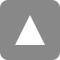# Department of Statistical Modeling

The Department of Statistical Modeling works on structural modeling of physical phenomena related to numerous factors, and conducts research on model-based statistical inference methodologies. By means of model-based prediction and control, modeling of complex systems, and data assimilation, the department aims to contribute to the development of modeling intelligence in many fields.

## Prediction and Control Group

The Prediction and Control Group works on the development and evaluation of statistical models, which function effectively in terms of prediction and control of phenomena, decision making, and scientific discoveries. These efforts involve data analysis and modeling related to phenomena that vary across time and space.

## Complex System Modeling Group

The Complex System Modeling Group conducts studies aimed at discovering the structures of complex systems, such as nonlinear systems and hierarchical networks, through statistical modeling. For these purposes, the group also considers Monte Carlo simulations, discrete mathematics, and computer science.

## Data Assimilation Group

The Data Assimilation Group works on the development of data assimilation techniques, which are procedures aimed at combining information derived from large amounts of observations and a numerical simulation model. By developing computational algorithms and high-performance parallel computing systems, the group aims to build a next-generation simulation model that can predict the future in real time.